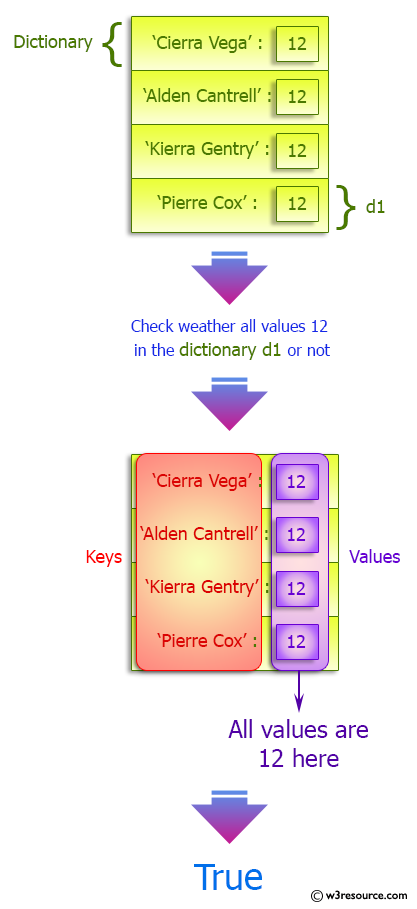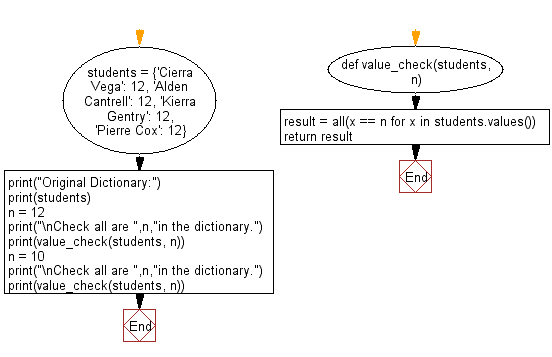﻿ Python: Check all values are same in a dictionary - w3resource# Python: Check all values are same in a dictionary

## Python dictionary: Exercise-45 with Solution

Write a Python program to check all values are same in a dictionary.

Sample Solution:

Python Code:

``````def value_check(students, n):
result = all(x == n for x in students.values())
return result

students = {'Cierra Vega': 12, 'Alden Cantrell': 12, 'Kierra Gentry': 12, 'Pierre Cox': 12}
print("Original Dictionary:")
print(students)
n = 12
print("\nCheck all are ",n,"in the dictionary.")
print(value_check(students, n))
n = 10
print("\nCheck all are ",n,"in the dictionary.")
print(value_check(students, n))
```
```

Sample Output:

```Original Dictionary:
{'Cierra Vega': 12, 'Alden Cantrell': 12, 'Kierra Gentry': 12, 'Pierre Cox': 12}

Check all are  12 in the dictionary.
True

Check all are  10 in the dictionary.
False
```

Pictorial Presentation:Flowchart:## Visualize Python code execution:

The following tool visualize what the computer is doing step-by-step as it executes the said program:

Python Code Editor:

Have another way to solve this solution? Contribute your code (and comments) through Disqus.

What is the difficulty level of this exercise?

Test your Programming skills with w3resource's quiz.

﻿

## Python: Tips of the Day

Invokes the provided function after ms milliseconds:

Example:

```from time import sleep

def tips_delay(fn, ms, *args):
sleep(ms / 1000)
return fn(*args)
print(tips_delay(
lambda x: print(x),
1000,
'w3r'
))
```

Output:

```w3r
None
```